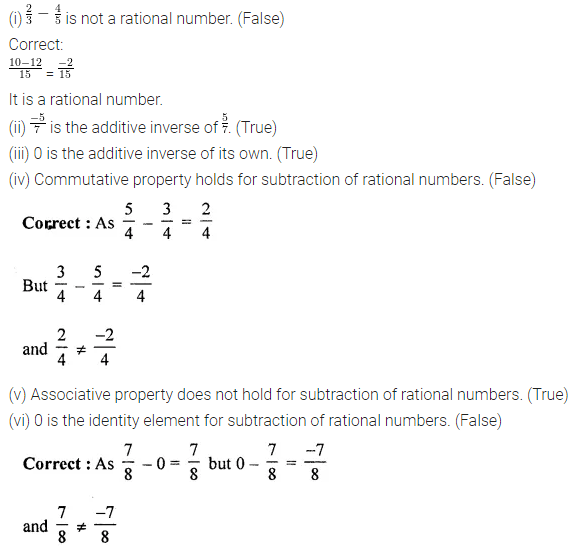# ML Aggarwal Class 8 Solutions for ICSE Maths Chapter 1 Rational Numbers Ex 1.2

## ML Aggarwal Class 8 Solutions for ICSE Maths Chapter 1 Rational Numbers Ex 1.2

Question 1.
Subtract:Solution: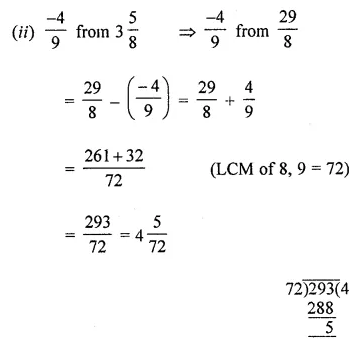Question 2.
Sum of two rational numbers is $$\frac { 3 }{ 5 }$$. If one of them is $$\frac { -2 }{ 7 }$$, find the other.
Solution:Question 3.
What rational number should be added to $$\frac { -5 }{ 11 }$$ to get $$\frac { -7 }{ 8 }$$?
Solution: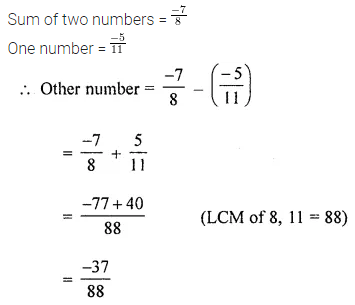Question 4.
What rational number should be subtracted from -4$$\frac { 3 }{ 5 }$$ to get -3$$\frac { 1 }{ 2 }$$ ?
Solution:Question 5.
Subtract the sum of $$\frac { -5 }{ 7 }$$ and $$\frac { -8 }{ 3 }$$ from the sum of $$\frac { 5 }{ 2 }$$ and $$\frac { -11 }{ 12 }$$.
Solution: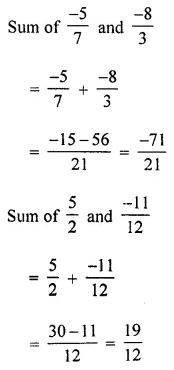Question 6.
If x = $$\frac { -4 }{ 7 }$$ and y = $$\frac { 2 }{ 5 }$$, then verify that x – y ≠ y – x.
Solution:Question 7.
If x = $$\frac { 4 }{ 9 }$$, y = $$\frac { -7 }{ 12 }$$ and z = $$\frac { -2 }{ 3 }$$, then verify that x – (y – z) ≠ (x – y) – z
Solution: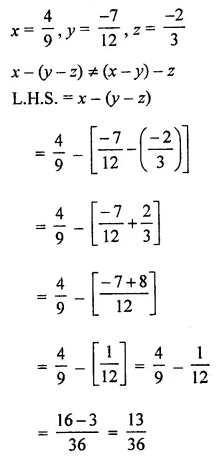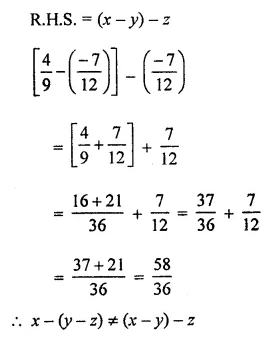Question 8.
Which of the following statement is true/fasle?
(i) $$\frac { 2 }{ 3 } -\frac { 4 }{ 5 }$$ is not a rational number.
(ii) $$\frac { -5 }{ 7 }$$ is the additive inverse of $$\frac { 5 }{ 7 }$$.
(iii) 0 is the additive inverse of its own.
(iv) Commutative property holds for subtraction of rational numbers.
(v) Associative property does not hold for subtraction of rational numbers.
(vi) 0 is the identity element for subtraction of rational numbers.
Solution: